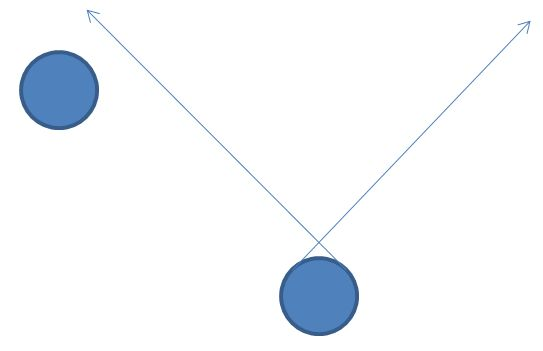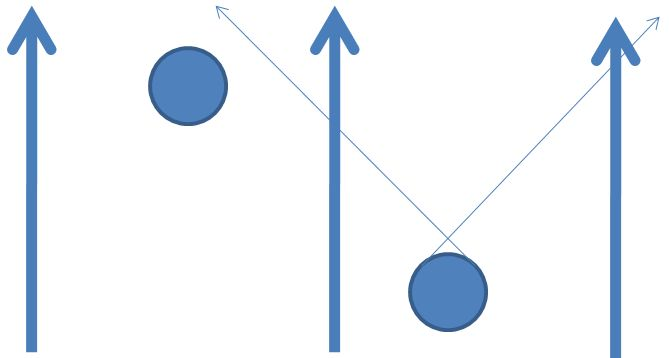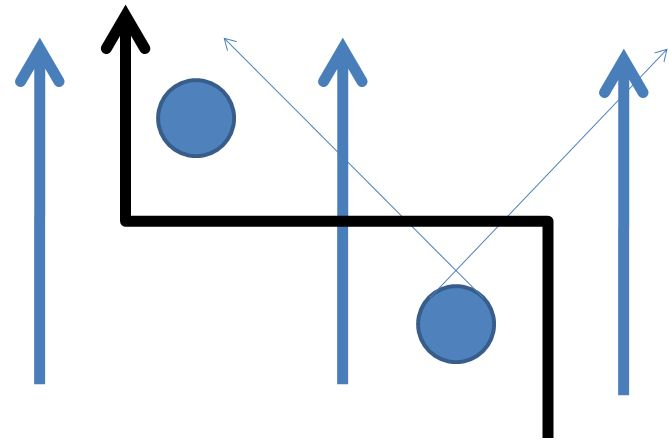# Eric Forgy directed spaceWarning: Under Construction

Given a directed graph $G$, a directed space is the space dual to the graded differential algebra (DGA)

$\Omega(G) = \bigoplus_{r\ge 0} \Omega^r(G)$

generated by $G$.

The space $\Omega^0(G)$ is spanned by the complete set of vertex projections

$\{\pi_v:G_0\to G_0|v\in G_0\}$

satisfying

(1)$\sum_{v\in G_0} \pi_v = 1.$

The commutative product on $\Omega^0(G)$ is given simply by composition

(2)$\pi_v \pi_{v'} = \delta_{v,v'} \pi_v.$

The space $\Omega^1(G)$ is spanned by elements

$\{\pi_e | e\in G_1\}$

with right multiplication $\Omega^1(G)\times\Omega^0(G)\to\Omega^1(G)$ given by

(3)$\pi_e\pi_v = \delta_{t(e),v} \pi_e$

and left multiplication $\Omega^0(G)\times\Omega^1(G)\to\Omega^1(G)$ given by

(4)$\pi_v\pi_e = \delta_{v,s(e)} \pi_e.$

### Generating a Discrete Space

The derivation

$d: \Omega^0(G)\to \Omega^1(G)$

applied to vertex projections results in,

(5)$\sum_{v\in G_0} d\pi_v = 0$

and

(6)$d(\pi_v \pi_{v'}) = (d \pi_v) \pi_{v'} + \pi_v (d \pi_{v'}) = \delta_{v,v'} d\pi_v,$

which, in particular, provides the graded commutative relation

(7)$[\pi_v,d\pi_v] = d\pi_v.$

Since $\{\pi_e|e\in G_1\}$ spans $\Omega^1(G)$, it follows that

(8)\begin{aligned} d\pi_v &= \sum_{e\in G_1} \alpha_e \pi_e, \alpha_e\in R \\ &= \sum_{e\in t^{-1}(v)} \alpha^{(t)}_e \pi_e + \sum_{e\in s^{-1}(v)} \alpha^{(s)}_e \pi_e\quad\text{from (3),(4), and (7),} \end{aligned}

which says $d\pi_v$ is a weighted sum of edges directed into $v$ and edges directed out of $v$.

Multiplying (6) by $\pi_v$ on the left and $\pi_{v'}$ on the right, results in

(9)$\pi_v (d\pi_{v'}) \pi_{v'} = (\delta_{v,v'} - 1) \pi_v (d\pi_v) \pi_{v'}$

#### Case: $v = v'$

When $v = v'$, combining (8) and (9) gives

(10)$\pi_v (d\pi_v) \pi_v = \sum_{e\in s^{-1}(v)\cap t^{-1}(v)} (\alpha^{(t)}_e + \alpha^{(s)}_e) \pi_e = 0,$

which implies that for loops

$\alpha^{(s)}_e = -\alpha^{(t)}_e.$

#### Case: $v \ne v'$

When $v \ne v'$, (8) gives

(11)$\pi_v (d\pi_v) \pi_{v'} = \sum_{e\in s^{-1}(v)\cap t^{-1}(v')} \alpha^{(s)}_e \pi_e$

and

(12)$\pi_v (d\pi_{v'}) \pi_{v'} = \sum_{e\in s^{-1}(v)\cap t^{-1}(v')} \alpha^{(t)}_e \pi_e$

which, when combined with (9), implies that

$\alpha^{(s)}_e = -\alpha^{(t)}_e.$

Therefore, (8) may be rewritten, in general, as

(13)$d\pi_v = \sum_{e\in t^{-1}(v)} \alpha_e \pi_e - \sum_{e\in s^{-1}(v)} \alpha_e \pi_e.$

and (12) may be rewritten as

(14)$\pi_v (d\pi_{v'}) \pi_{v'} = \sum_{e\in s^{-1}(v)\cap t^{-1}(v')} \alpha_e \pi_e.$

Question: Could it be that $\alpha_e$ is related to the Leinster measure?

Note also that (setting $\alpha_e = 1$ and assuming no loops)

(15)$\sum_{v,v'} \pi_v (d\pi_{v'}) \pi_{v'} = \sum_{v'} (d\pi_{v'}) \pi_{v'} = \sum_{e} \pi_e.$

or

(16)$\sum_{e} \pi_e = \sum_{v} (d\pi_{v}) \pi_{v},$

which allows us to convert a sum over all edges to a sum over all nodes.

category: maths

Revised on October 3, 2010 at 14:12:29 by Eric Forgy